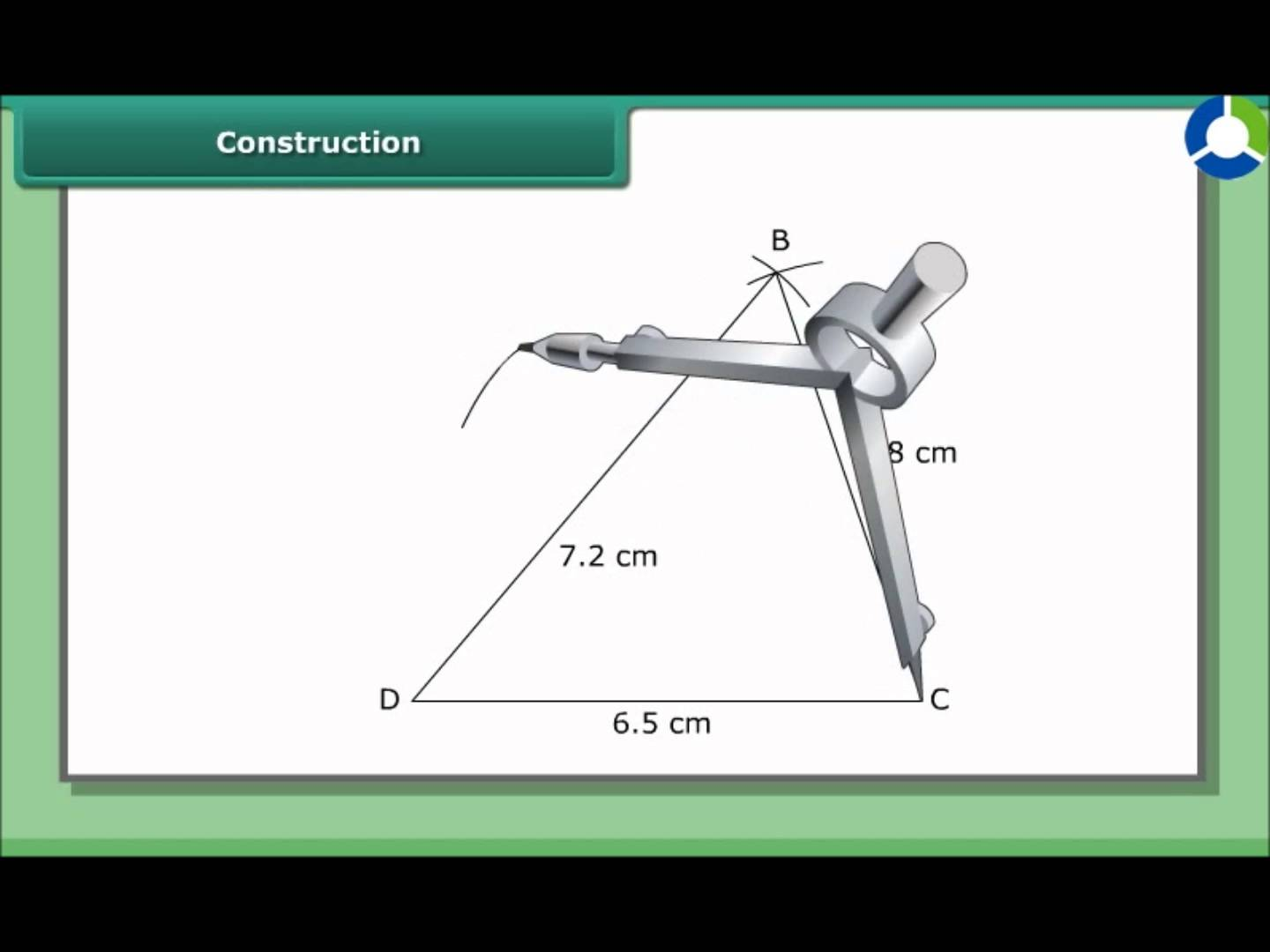# Practical Geometry worksheet for class 6## myCBSEguide App

CBSE, NCERT, JEE Main, NEET-UG, NDA, Exam Papers, Question Bank, NCERT Solutions, Exemplars, Revision Notes, Free Videos, MCQ Tests & more.

## Symmetry worksheet for class 6 Important Topics

• Introduction
• The Circle
• A Line Segment
• Perpendiculars
• Angles## Some important Facts about Practical Geometry worksheet for class 6

1. This chapter deals with methods of drawing geometrical shapes. 1. We use the following mathematical instruments to construct shapes:
2. The compasses
3. The divider
4. Set-squares
5. The protractor
2. Using the ruler and compasses, the following constructions can be made:
1. A circle, when the length of its radius is known.
2.  A line segment, if its length is given.
3. A copy of a line segment.
4. A perpendicular to a line through a point (a) on the line (b) not on the line.

## NCERT class 6  Mathematics Solved Worksheets

• Chapter 1 – Knowing Our Numbers
• Chapter 2 – Whole Numbers
• Chapter 3 – Playing with Numbers
• Chapter 4 – Basic Geometrical Ideas
• Chapter 5 – Understanding Elementary Shapes
• Chapter 6 – Integers
• Chapter 7 – Fractions
• Chapter 8 – Decimals
• Chapter 9 – Data Handling
• Chapter 10 – Mensuration
• Chapter 11 – Algebra
• Chapter 12 – Ratio and Proportion
• Chapter 13 – Symmetry
• Chapter 14 – Practical Geometry

## Perpendiculars

You know that two lines (or rays or segments) are said to be perpendicular if they intersect such that the angles formed between them are right angles. In the figure, the lines l and m are perpendicular. Mathematics 280 The corners of a foolscap paper or your notebook indicate lines meeting at right angles.

To download Printable worksheets for class 6 Mathematics and Science; do check myCBSEguide app or website. myCBSEguide provides sample papers with solution, test papers for chapter-wise practice, NCERT solutions, NCERT Exemplar solutions, quick revision notes for ready reference, CBSE guess papers and CBSE important question papers. Sample Paper all are made available through the best app for CBSE students and myCBSEguide website.